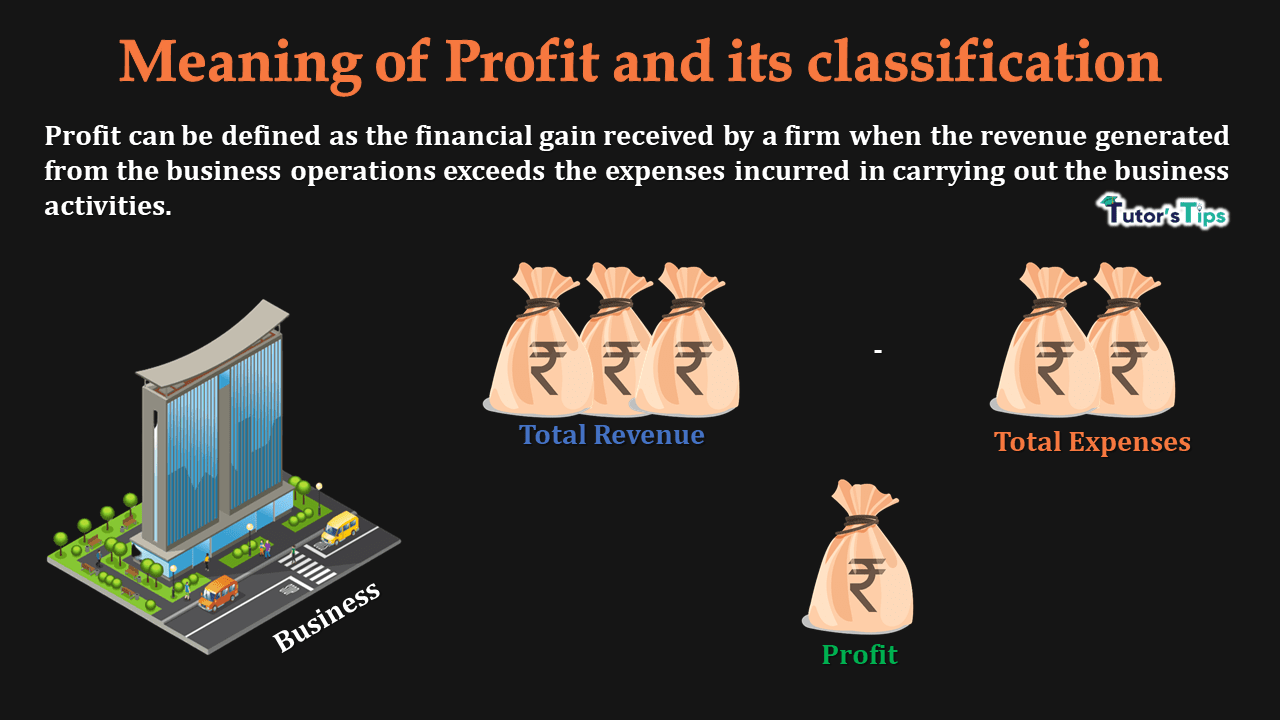# Meaning of Profit and its classificationMeaning of Profit and its classification-min

Meaning of profit includes the money remained to an enterprise after settling all the expenses in the business.

## Meaning of Profit :

The meaning of profit in a business firm refers to the excess of revenue over costs over a period of time. In other words, profit can be defined as the financial gain received by a firm when the revenue generated from the business operations exceeds the expenses incurred in carrying out the business activities.

### Example explaining the meaning of profit:

Suppose, a business firm earned revenue of Rs.60,00,000 from business operations over a year and incurred expenditure worth Rs. 50,00,000. Thus, the profit would be:

Profit = Total Revenue – Total Costs

= 60,00,000-50,00,000

= Rs. 10,00,000

Therefore, profit can be defined as the difference between total revenue and total costs. But, it is here important to note that when revenue is greater than costs, there would be a profit. On the other hand, when total revenue is less than total costs, there would be a loss.

## Classification of Profit:

The profit can be classified as :

On the basis of Usage:

• Accounting Profits
• Economic Profits :
• Gross Profits
• Net Profits

On the basis of performance:

• Abnormal Profits
• Normal Profits
• Sub Normal Profits

### On the basis of usage:

#### 1. Accounting Profit:

It refers to the difference between total revenue and total costs. But, here total costs consist of only explicit costs. It can be written as:

Accounting Profit = TR- TC (includes only explicit costs)

Thus, the accountants consider only accounting profit while estimating the income of a business.

#### 2. Economic Profit:

It refers to the difference between total revenue and total costs where Total cost includes both explicit as well as implicit costs). It can be written as:

Economic Profit = TR -TC including implicit and explicit costs

In economics, we focus only on economic profits as it shows the real profitability of any business enterprise. Therefore, economic profits are considered by economists.

The economic profits can further be estimated on the basis of gross profits and net profits:

##### a) Gross Profit:

Gross profit refers to the difference between total revenue and total variable cost. In other words, the profit obtained by deducting only variable costs from the total revenue is known as Gross Profit. Also, It can be written as:

Gross Profit = Total Revenue – Total Variable Cost

or

Gross Profit = TR – TVC

##### a) Net Profit:

It refers to the difference between Total revenue and Total Costs. In other words, when we deduct total variable and total fixed costs both from the total revenue, the amount we get is known as net profit. Hence, it can be written as:

Net Profit = TR- (TVC+TFC)

Or

Net profit = TR – TC

Here, TR refers to total revenue

TVC refers to total variable cost

TFC denotes Total fixed cost

TC denotes the total cost

### On the basis of Performance:

In economics, the performance of a business is assessed with reference to three levels of profits:

#### 1. Abnormal Profit:

A producer or producing firm earns abnormal profits when Total revenue exceeds total costs in the business. In other words,

There is abnormal profit, when:

TR > TC

or

TR/Q >TC/Q

or

AR>AC

Therefore, when in any business, The average revenue is greater than the average costs, there would be abnormal profits. Hence, these profits are also known as Extra-normal profits.

#### 2. Normal Profit:

A producer or producing firm earns normal profits when Total revenue is equal to total costs in the business. In other words,

There is a normal profit, when:

TR = TC

or

TR/Q =TC/Q

or

AR = AC

Therefore, when in any business, The average revenue equals the average costs, there would be normal profits.

In the case of normal profits, profits are a part of total costs. Normal profits can be defined as the minimum return, a producer expects from its investment. If there is no normal profit, the investor or producer can shift its capital investment to somewhere else.

#### 3. Sub-normal Profit:

A producer or producing firm earns sub-normal profits when Total costs exceed total revenue in the business. In other words,

There is abnormal profit, when:

TR < TC

or

TR/Q <TC/Q

or

AR < AC

Therefore, when in any business, The average revenue is less than the average costs, there would be sub-normal profits. Hence, these profits are also known as Losses.

Comment if you have any questions.

References: